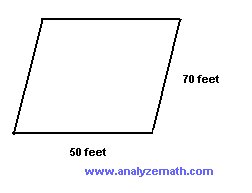Figure B is inside figure A shown below. Which statement is correct?. The perimeter of A is equal to the perimeter of B The perimeter of A is smaller than the perimeter of B The perimeter of A is larger than the perimeter of B cannot answer the question Find the perimeter of the rectangle shown below.. 21 cm 108 cm 216 cm 42 cm Find the perimeter of the square shown below.. 60 m 225 m 120 m 30 m Find the perimeter of the shape below.. 42 mm 70 mm 180 mm 56 mm What is the perimeter of the parallelogram shown below?. 120 feet 3,500 feet 7,000 feet 240 feet What is the perimeter of a an equilateral triangle of side 20 cm? 80 cm 60 cm 400 cm 40 cm A plane figure has 5 congruent sides (same size). The perimeter of this figure is equal to 600 meters. Find the length of one side of this figure. 120 centimeters 3000 meters 120 meters 150 meters In the figure below, a large rectangle has a length of 60 meters and a width of 20 meters. A smaller rectangle has a length of 30 meters and width of 20 meters. Which statement is correct?. The perimeter of the large rectangle is twice the perimeter of the small rectangle. The perimeter of the large rectangle is equal to the perimeter of the small rectangle. The perimeter of the large rectangle is 30 meters more than the perimeter of the small rectangle. The perimeter of the large rectangle is equal to 160 meters and the perimeter of the small rectangle is equal to 100 meters. The perimeter of a square is equal to 88 feet. What is the length of the side of this square? 22 feet 44 feet 11 feet 352 feet The perimeter of the square is twice the perimeter of the triangle. What is the length of the side of the square?. 15 in 7.5 in 60 in 30 in Answers to the Above Questions C D A B D B C D A A false A